streamslice

Plot streamlines in slice planes

•Description

example

streamslice(X,Y,Z,U,V,W,xslice,yslice,zslice) draws automatically spaced streamlines from 3-D vector data U, V, and W. Specify X, Y, and Z as the coordinate data. Specify xslice, yslice, and zslice as the slice locations orthogonal to a particular axis.

streamslice(U,V,W,xslice,yslice,zslice) uses the default coordinate data for U, V, and W. The (x,y,z) location for each element in U, V, and W is based on the column, row, and page index, respectively.

example

streamslice(X,Y,U,V)draws automatically spaced streamlines from 2-D vector data U and V. Specify X and Y as the coordinate data.

example

streamslice(U,V) uses the default coordinate data for U and V. The (x,y) location for each element in U and V is based on the column and row index, respectively.

example

streamslice(___,arrowsmode), where arrowsmode is 'noarrows', does not draw direction arrows. The default for arrowsmode is 'arrows', which draws direction arrows. Use this option with any of the input arguments from the previous syntaxes.

example

streamslice(___,density) modifies the automatic spacing of the streamlines, where density is a positive scalar. The default value is 1. Higher values produce more streamlines on each plane. For example, 2 produces approximately twice as many streamlines as the default, while 0.5 produces approximately half as many.

streamslice(___,method) specifies the interpolation method, where method can be 'linear', 'cubic', or 'nearest'.

streamslice(ax,___) plots into the specified axes, instead of into the current axes object (gca).

example

lineobj = streamslice(___) returns the Line objects created.

example

[verts,averts] = streamslice(___) returns vertices for drawing the streamlines and arrows as cell arrays. This syntax does not plot the streamlines.

Examples

collapse all

Load the wind dataset, which loads the 3-D arrays u, v, w, x, y, and z, representing air currents over regions of North America. Draw streamlines along the slice plane where z = 5.

streamslice(x,y,z,u,v,w,[],[],5)
axis tight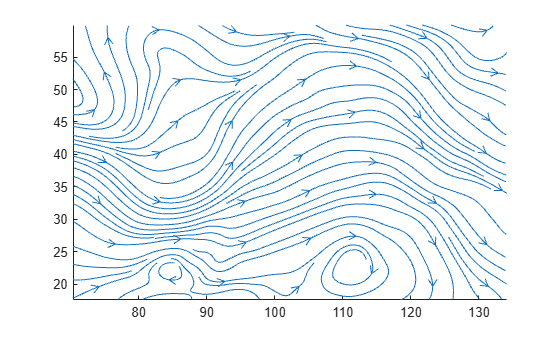Load the wind dataset and draw streamlines without direction arrows along the slice plane where z = 5.

streamslice(x,y,z,u,v,w,[],[],5,'noarrows')
axis tight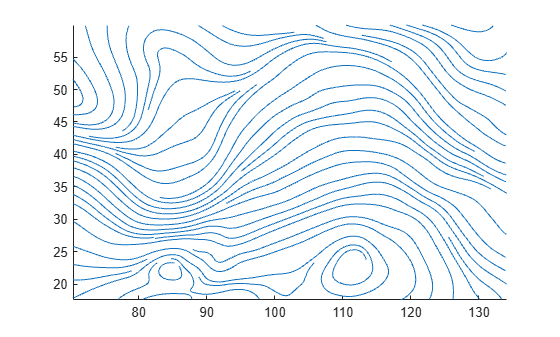Load the wind dataset and draw streamlines along the slice plane, where z = 5. Use a density value of 2 to draw approximately twice as many streamlines as the default.

streamslice(x,y,z,u,v,w,[],[],5,2)
axis tightLoad the wind dataset and compute the vertices of streamlines along the slice planes, where z = 1, 5, and 10.

[verts, ~] = streamslice(x,y,z,u,v,w,[],[],[1,5,10]);

Pass the vertices to streamtube to visualize the lines as tubes.

streamtube(verts,1)

view(3)
axis tight
camlight
lighting gouraudPlot streamlines and return the line objects in l.

[x,y] = meshgrid(-10:10);
u = 2.*x.*y;
v = y.^2 - x.^2;
l = streamslice(x,y,u,v);
axis tightSet the line width of the streamlines to 2. Set their color to red. Use the set function to change the properties of all the lines at the same time.

set(l,'LineWidth',2)
set(l,'Color','r');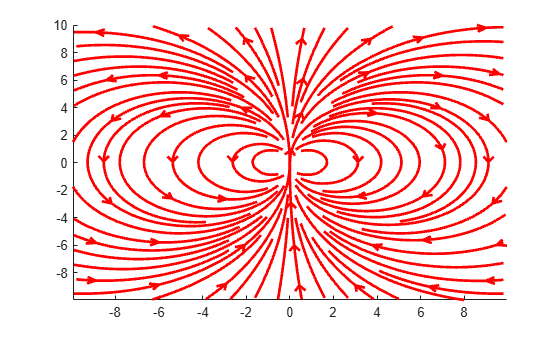First, create a surface plot.

z = peaks;
surf(z);Compute the two-dimensional gradient of z. Use the x and y components as the vector data input for streamslice. Set the color of the streamlines to black.

l = streamslice(u,v);
set(l,'Color','k');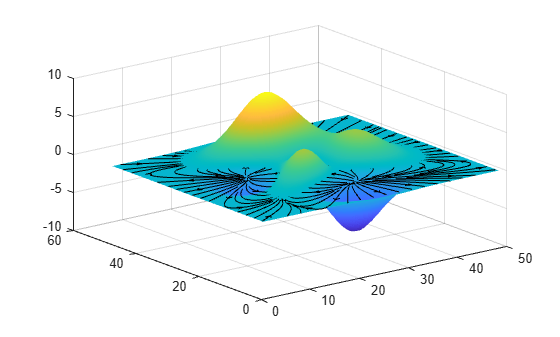Displace the streamlines on the z-axis so that they are drawn on the surface of the curve.

for i=1:length(l)
zi = interp2(z,l(i).XData, l(i).YData);
l(i).ZData = zi;
end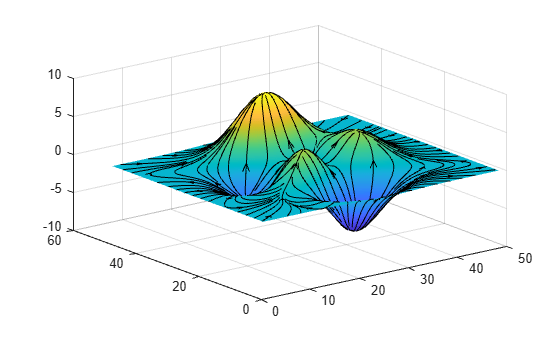Input Arguments

collapse all

x-axis coordinate data, specified as a 2-D or 3-D array. It must be monotonic, but does not need to be uniformly spaced.

X, Y, Z, U, V, and W must be the same size.

You can use the meshgrid function to create X, Y, and Z matrices.

y-axis coordinate data, specified as a 2-D or 3-D array. It must be monotonic, but does not need to be uniformly spaced.

X, Y, Z, U, V, and W must be the same size.

You can use the meshgrid function to create X, Y, and Z matrices.

z-axis coordinate data, specified as a 3-D array. It must be monotonic, but does not need to be uniformly spaced.

X, Y, Z, U, V, and W must be the same size.

You can use the meshgrid function to create X, Y, and Z matrices.

x-components of vector data, specified as a 2-D or 3-D array.

X, Y, Z, U, V, and W must be the same size.

y-components of vector data, specified as a 2-D or 3-D array.

X, Y, Z, U, V, and W must be the same size.

z-components of vector data, specified as a 3-D array.

X, Y, Z, U, V, and W must be the same size.

x-axis slice values, specified in one of these forms:

• Scalar — Draw one slice plane orthogonal to the x-axis at the specified location.

• Vector — Draw multiple slice planes orthogonal to the x-axis at the specified locations.

• [] — Do not draw any slice planes.

y-axis slice values, specified in one of these forms:

• Scalar — Draw one slice plane orthogonal to the y-axis at the specified location.

• Vector — Draw multiple slice planes orthogonal to the y-axis at the specified locations.

• [] — Do not draw any slice planes.

z-axis slice values, specified in one of these forms:

• Scalar — Draw one slice plane orthogonal to the z-axis at the specified location.

• Vector — Draw multiple slice planes orthogonal to the z-axis at the specified locations.

• [] — Do not draw any slice planes.

Streamline density, specified as a positive scalar.

The default value is 1. Higher values produce more streamlines on each plane. For example, 2 produces approximately twice as many streamlines as the default, while 0.5 produces approximately half as many.

Arrow display mode, specified as one of these values:

• 'arrows' — Draw direction arrows on the streamlines.

• 'noarrows' — Do not draw direction arrows on the streamlines.

Interpolation method, specified as one of these values:

• 'linear' — Linear interpolation of the values at neighboring grid points in each respective dimension.

• 'cubic' — Cubic interpolation of the values at neighboring grid points in each respective dimension.

• 'nearest' — Nearest grid point value.

Target axes, specified as an Axes object. If you do not specify the axes, then streamslice plots into the current axes.

Output Arguments

collapse all

Line objects for streamlines and direction arrows, returned as an array.

Streamline vertices, returned as a cell array. Each element of the cell array is a matrix of vertices for one line.

You can pass these values to any of the streamline drawing functions (streamline, streamribbon, streamtube).

Arrow vertices, returned as a cell array. Each element of the cell array is a matrix of vertices for one arrow. If arrowsmode is 'noarrows', averts is an empty cell array.

You can pass these values to streamline.